# Preface

This section presents linearization technique to analyze stability of critical points of nonlinear systems of differential equations.

Introduction to Linear Algebra with Mathematica

The EquationTrekker package is a great package for plotting and exploring phase portraits

# Almost Linear Systems

There is a broad class of dynamic systems solutions of which can be analysed based on linear systems of differential equations. In what follows we analyze auttonomous system of equation
\begin{equation} \label{EqLinear.1} \frac{{\text d}{\bf x}(t)}{{\text d}t} = {\bf f}\left( {\bf x}(t) \right) \end{equation}
near isolated critical points where f(x) = 0. Recall that an equilibrium solution x(t) = x* is isolated if in some its neighborhood there is no other critical points except x*.
Consider the following system:
\begin{equation} \label{EqLinear.2} \frac{{\text d}{\bf x}(t)}{{\text d}t} = {\bf A}\,{\bf x}(t) + {\bf g}\left( {\bf x} \right) , \end{equation}
and suppose that x = 0 is an isolated critical point of Eq.\eqref{EqLinear.2}. This system is called an almost linear system (or local linear) in the neighborhood of x = 0 if
• g(x) is an analytic function at the origin (or, more precise, admits the second order Taylor's approximation);
• as x0,
\begin{equation} \label{EqLinear.3} \frac{\| {\bf g}({\bf x}) \|}{\| {\bf x} \|} \rightarrow 0 , \qquad \mbox{where} \quad \| {\bf x}\| = \left( x_1^2 + x_2^2 + \cdots + x_n^2 \right)^{1/2} . \end{equation}
Relation \eqref{EqLinear.3} guarantees immediately that the origin is an isolated critical point. Since function g(x) is small compares to x in a neighborhood of the critical point, it can be treated as a pertubation to the corresponding linear system $$\dot{\bf x} = {\bf A}\,{\bf x} .$$ Most practical systems are of type \eqre{EqLinear.2} because the so-called linear laws of physics that are involved in deriving the equations of motions are, in fact, not linear. The linear formulation of Hooke's or Ohm's law for instance is an idealization. The derivations from the linear model are in general "small" and therefore the assumption \eqref{EqLinear.2} is justified within certain bounds. Of course, we must then also in general restrict the dependent variable to "small" values.

Planar Systems

Theorem 1: If functions f(x, y) and g(x, y) in planar system
\begin{equation} \label{EqLinear.4} \dot{x} = f(x,y) , \qquad \dot{y} = g(x,y) \end{equation}
admits a second order Taylor's polynomial approximation in the neighborhood of the critical point (x0, y0), then system \eqref{EqLinear.4} is almost linear.
In planar case, we have almost linear system
\begin{equation} \label{EqLinear.5} \begin{cases} \dot{x} = a\,x + b\,y + f(x,y) , \\ \dot{y} = c\,x + d\,y + g(x,y) , \end{cases} \end{equation}
where 𝑎, b, c, d are real constants, with 𝑎d - cb ≠ 0 (this condition guarantees that the origin is an isolated critical point), and f = f(x, y), g = g(x, y) are real continuous functions defined in some neighborhood of the origin. The functions f, g are called pertubations, and the nonlinear system \eqref{EqLinear.5} is called pertubed system corresponding to the linear system
\begin{equation} \label{EqLinear.6} \begin{cases} \dot{x} = a\,x + b\,y , \\ \dot{y} = c\,x + d\,y , \end{cases} \qquad \mbox{or} \qquad \frac{\text d}{{\text d}t} \begin{bmatrix} x(t) \\ y(t) \end{bmatrix} = \begin{bmatrix} a&b \\ c&d \end{bmatrix} \begin{bmatrix} x(t) \\ y(t) \end{bmatrix} . \end{equation}
The nonlinear system \eqref{EqLinear.5} is almost linear if
$f = o(r), \qquad g=o(r) \qquad \mbox{as} \quad r = \sqrt{x^2 + y^2} \to 0^{+} .$
Note that the continuity condition of functions f(x, y), g(x, y) is not sufficient to guarantee the uniqueness of the initial value problem for nonlinear system \eqref{EqLinear.5}.

Example 1: We consider the planar system with non-holomorphic slope functions:
$\dot{x}= -y\,\sqrt{x^2 + y^2} , qquad \dot{y}= x\,\sqrt{x^2 + y^2}$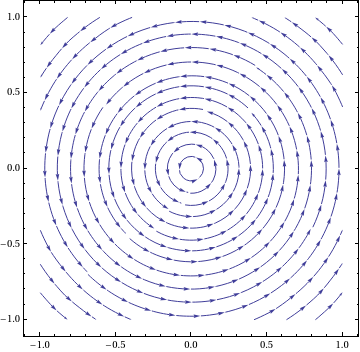However, the origin is a stable center. In fact, the solution paths of it are described in polar coordinates by $\dot{r} = 0 , \qquad \dot{\theta} = r .$ Thus, $r = c_1 , \qquad \theta = c_1 t + c_2 ,$ with some constants c1 and c2. StreamPlot[{-y*Sqrt[x^2 + 1* y^2], x*Sqrt[1*x^2 + y^2]}, {x, -1, 1}, {y, -1, 1}, PerformanceGoal -> "Quality", StreamPoints -> 35, PlotTheme -> "Classic"] Figure 1: Phase portrait. Mathematica code

■

To analyze the trajectories of Eq.\eqref{EqLinear.5}, it is convenient to use polar coordinates

$x = r\,\cos\theta , \qquad y = r\,\sin \theta .$
Taking the derivative of the square of the radius, we get
\begin{align*} \frac{1}{2}\,\frac{\text d}{{\text d}t} \left( r^2 \right) &= r\,\dot{r} = \frac{1}{2}\, \frac{\text d}{{\text d}t} \left( x^2 + y^2 \right) = x\,\dot{x} + y\,\dot{y} \\ &= r\,\cos\theta \left[ ar\,\cos\theta + br\,\sin\theta + f \right] + r\,\sin\theta \left[ cr\,\cos\theta + dr\,\sin\theta + g \right] . \end{align*}
Cancelling r, we obtain
\begin{equation} \label{EqLinear.7} \frac{{\text d}r}{{\text d}t} = r \left[ a\,\cos^2 \theta + \left( b+c \right) \cos\theta \,\sin\theta + d\,\sin^2 \theta \right] + \cos\theta \,F(r, \theta ) + \sin\theta\,G(r, \theta ) , \end{equation}
where
$F(r, \theta ) = f\left( r\,\cos\theta , r\,\sin\theta \right) , \qquad G(r, \theta ) = g\left( r\,\cos\theta , r\,\sin\theta \right) .$
Similarly, we differentiate tanθ:
\begin{align*} \frac{\text d}{{\text d}t} \left( \tan\theta \right) &= \frac{1}{\cos^2 \theta} \, \frac{{\text d}\theta}{{\text d}t} = \frac{\text d}{{\text d}t} \left( \frac{y}{x} \right) = \frac{x\,\dot{y} - y\,\dot{x}}{x^2} . \end{align*}
Multiplying both sides by x², we obtain
\begin{equation} \label{EqLinear.8} r\, \frac{{\text d}\theta}{{\text d}t} = r\, \left[ c\,\cos^2 \theta + \left( d-a \right) \cos\theta \,\sin\theta - b\, \sin^2 \theta \right] + \cos\theta \,G(r, \theta ) - \sin\theta \,F(r, \theta ) , \end{equation}
If φ = ⟨ φ₁, φ₂ ⟩ is a solution of the nonlinear system \eqref{EqLinear.4}, the polar functions
$\rho (t) = \sqrt{\phi_1^2 (t) + \phi_2^2 (t)} , \qquad \theta (t) = \arctan \frac{\phi_2 (t)}{\phi_1 (t)}$
constitute a solution of the polar equations \eqref{EqLinear.7}, \eqref{EqLinear.8}.

If there exists a δ: 0 < δ ≤ r₀, such that, for any solution path φ = ⟨ φ₁, φ₂ ⟩ of nonlinear system \eqref{EqLinear.4} that has at least one point inside the circle 0 < r < δ, the solution exists over a t half line, and if

$\phi = \langle \phi_1 (t), \psi_2 (t) \rangle \to (0,0)$
as t → ∞, then the origin is called an attractor (or sink) for the nonlinear system. If the above limit holds for t → −∞, then the origin is called a repeller (or sourse).

Example 1: Consider the nonlinear system
$\frac{{\text d}x}{{\text d}t} = -y + x \left( x^2 + y^2 \right) , \qquad \frac{{\text d}y}{{\text d}t} = x - y \left( x^2 + y^2 \right)$
and its linear approximation
$\dot{x} = -y , \qquad \dot{y} = x .$
The origin is the center for the linear system and it is stable because the corresponding matrix
$\begin{bmatrix} 0&-1 \\ 1 & 0 \end{bmatrix}$
is not singular and have two pure imaginary eigenvalues λ = ±j.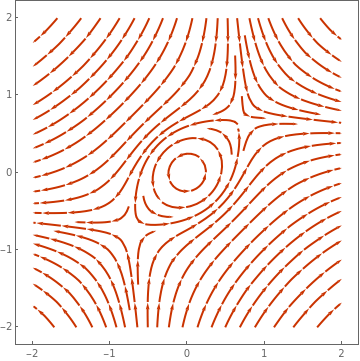However, the origin is a stable center. In fact, the solution paths of it are described in polar coordinates by $\dot{r}^2 = -2\,r^4 , \qquad \dot{\theta} = 1 .$ Thus, $r^2 = \frac{r_0^2}{1 + 2t\,r_0} , \qquad \theta = \theta_0 + t .$ StreamPlot[{-y + x*(x^2 + y^2), x - y*(x^2 + y^2)}, {x, -2, 2}, {y, -2, 2}, PerformanceGoal -> "Quality", PlotTheme -> "Web"] Figure 1: Phase portrait. Mathematica code

A similar problem
$\frac{{\text d}x}{{\text d}t} = -y + x \left( x^2 + y^2 \right) , \qquad \frac{{\text d}y}{{\text d}t} = x + y \left( x^2 + y^2 \right)$
has the same linearization, but the solutions behave differently.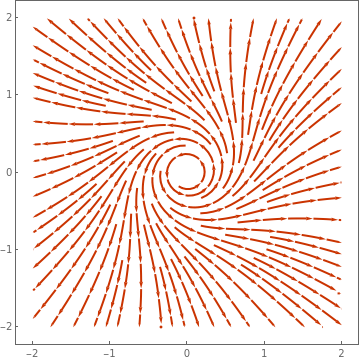However, the origin is an unstable center. In fact, the solution paths of it are described in polar coordinates by $\dot{r}^2 = 2\,r^4 , \qquad \dot{\theta} = 1 .$ Thus, $r^2 = \frac{r_0^2}{1 + 2t\,r_0} , \qquad \theta = \theta_0 + t .$ StreamPlot[{-y + x*(x^2 + y^2), x + y*(x^2 + y^2)}, {x, -2, 2}, {y, -2, 2}, PerformanceGoal -> "Quality", PlotTheme -> "Web"] Figure 2: Phase portrait. Mathematica code

A similar problem
$\frac{{\text d}x}{{\text d}t} = -y - x \left( x^2 + y^2 \right) , \qquad \frac{{\text d}y}{{\text d}t} = x - y \left( x^2 + y^2 \right)$
has the same linearization, but the solutions behave differently.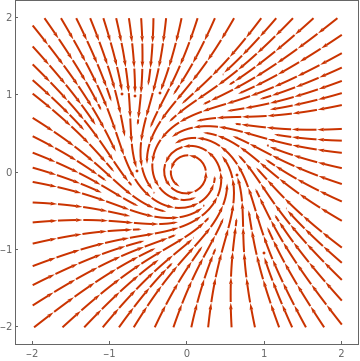However, the origin is an asymptotically stable center. In fact, the solution paths of it are described in polar coordinates by $\dot{r}^2 = 2\,r^4 , \qquad \dot{\theta} = 1 .$ Thus, $r^2 = \frac{r_0^2}{1 + 2t\,r_0} , \qquad \theta = \theta_0 + t .$ StreamPlot[{-y + x*(x^2 + y^2), x + y*(x^2 + y^2)}, {x, -2, 2}, {y, -2, 2}, PerformanceGoal -> "Quality", PlotTheme -> "Web"] Figure 3: Phase portrait. Mathematica code

■
Example 2: Consider the system
$\begin{split} \dot{x} &= -a\,x, \\ \dot{y} &= y \left[ \sin \left( \ln t \right) + \cos \left( \ln t \right) \right] + x^2 , \end{split}$
where
$\frac{1}{2} < a < \left( 1 + e^{-\pi} \right) /2 \approx 0.521607 , \quad t> 0 .$
All solutions are given by
$\begin{split} x &= x(t) = c_2 e^{-at} , \\ y &= y(t) = e^{t\,\sin (\ln t) - 2at} \left( c_2 + c_1^2 \int_0^t e^{-s\.\sin (ln s)} {\text d}s \right) , \end{split}$
with some constants c1 and c2. We first underestimate the integral
$F(t) = \int_0^t e^{-f(s)} {\text d}s , \qquad f(t) = t\,\sin \left( \ln t \right) .$
Note that if 0 < u < v < t, then
$F(t) > \int_u^v e^{-f(s)} {\text d}s$
Next, we find an interval (u, v) on which f(s) is decreasing. We have
$f' (s) = \sin \sigma + \cos \sigma , \qquad \mbox{where} \quad \sigma = \ln s .$
Function f(s) is decreasing for
$\frac{3\pi}{4} + 2n\pi \le \ln s \le \frac{7\pi}{4} + 2n\pi ,$
or
$e^{\frac{3\pi}{4} + 2n\pi} \le s \le e^{\frac{7\pi}{4} + 2n\pi} , \quad n\ge 0.$p1 = Plot[{Cos[x], -Sin[x]}, {x, 0, 2*Pi}, Filling -> {1 -> {2}}, FillingStyle -> {Green, White}, PlotTheme -> "Web", Ticks -> {{0, 3 Pi/4, 7*Pi/4}, {-1, 1}}]; arx = Graphics[{Arrowheads[0.06], Thick, Arrow[{{-0.1, 0}, {6.45, 0}}]}]; t1 = Graphics[{Black, Text[Style["3$Pi]/4", 18], {2.3, -0.2}]}]; l1 = Graphics[Line[{{2.3, 0}, {2.3, 0.1}}]]; t2 = Graphics[{Black, Text[Style["7\[Pi]/4", 18], {5.5, -0.2}]}]; l2 = Graphics[Line[{{5.5, 0}, {5.5, 0.1}}]]; cos = Graphics[{Black, Text[Style["cos(\[Sigma])", 18], {1.5, 0.7}]}]; sin = Graphics[{Black, Text[Style["-sin(\[Sigma])", 18], {1.2, -0.5}]}]; Show[p1, arx, t1, l1, t2, l2, cos, sin] Let \[ t_n = e^{\pi /2 + 2n\pi} , \quad u_n = t_n e^{-\pi} , \quad v_n = t_n e^{-3\pi /4} , \qquad n=1,2,\ldots .$
Then
$F \left( t_n \right) \ge \int_{u_n}^{v_n} e^{-f(s)} {\text d} s \ge \left( v_n - u_n \right) e^{-f(u_n )} = \left( v_n - u_n \right) e^{u_n} .$
Hence,
\begin{align*} y\left( t_n \right) &\ge e^{t_n \sin (\ln t_n ) - 2a\,t_n} \left\{ c_2 + c_1^2 \int_{u_n}^{v_n} e^{-f(s)} {\text d} s \right\} \\ &\ge e^{t_n \left( 1 - 2a \right)} \left\{ - \left\vert c_2 \right\vert + c_1^2 \left( e^{-3\pi /4} - e^{-\pi} \right) t_n \exp \left( t_n e^{-\pi} \right) \right\} \\ &= - \left\vert c_2 \right\vert e^{t_n \left( 1 - 2a \right)} + c_1^2 \left( e^{-3\pi /4} - e^{-\pi} \right) t_n \exp \left\{ t_n \left( 1 + e^{-\pi} - 2a \right) \right\} . \end{align*}
Then for any choice of c2 and c1 ≠ 0, we have
$\lim_{n\to\infty} \sup y\left( t_n \right) = \infty .$
Therefore, the given almost linear system of ODEs has a critical point at the origin which is unstable. However, the linear approximation
$\begin{split} \dot{x} = -a\,x , \\ \dot{y} = y \left[ \sin (\ln t) + \cos (\ln t) - 2a \right] \end{split}$
has a fundamental matrix solution
$\begin{bmatrix} e^{-at} & 0 \\ 0 & e^{t\,\sin (\ln t) - 2at} \end{bmatrix}$
approaches zero as t → ∞. So the origin of the linearized system is globally asymptotically stable.    ■

# Linearization

the first question that arrises from the almost linear system \eqref{EqLinear.2} is whether the stability behavior of the linear system implicitly defined in \eqref{EqLinear.2} is "sensitive", i.e., whether and to what extent it is affected by the nonlinear terms. To answer this question we usually introduce a further idealization namely, we assume that vector function g(x) has Taylor series expansions beginning with second or higher degree terms. However, before jumping into the linearization procedure, we present some examples to develop an intuitive approach.
Example 2: Consider a nonlinear system
$\dot{x} = x+y -x^2 , \qquad \dot{y} = y - x^3 .$
The given system has the only one real critical point---the origin.
Solve[{x + y - x^2 == 0, y - x^3 == 0}, {x, y}, Reals]
{{x -> 0, y -> 0}}
The linear approximation is obvious
$\dot{x} = x+y , \qquad \dot{y} = y \qqiad\Longrightarrow \qquad {\bf A} = \begin{bmatrix} 1& 1 \\ 0&1 \end{bmatrix} .$
Now we plot the phase portrait for both, the given autonoous system and for its linearization.
StreamPlot[{x + y - x^2, y - x^3}, {x, -1, 1}, {y, -1, 1}, PerformanceGoal -> "Quality", PlotTheme -> "Classic"]
StreamPlot[{x + y, y}, {x, -1, 1}, {y, -1, 1}, PerformanceGoal -> "Quality", PlotTheme -> "Classic"]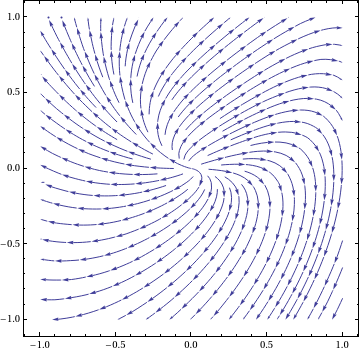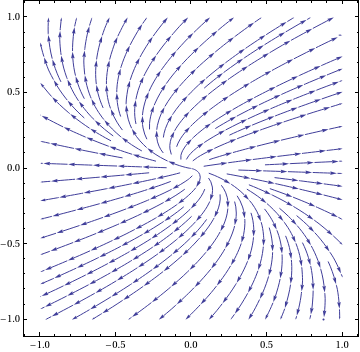Phase portrait for nonlinear system. Phase portrait for linear approximation.

■
Example 2: Consider a nonlinear system
$\dot{x} = x+y^2 , \qquad \dot{y} = -y .$
f[x_, y_] = {x + y^2, -y};
Print["f(x,y) = ", MatrixForm[f[x, y]]]
A = Table[D[f[x, y][[i]], {x, y}[[j]]], {i, 2}, {j, 2}] /. {x -> 0,
y -> 0};
{d, v} = Eigensystem[A];
T = Transpose[v];
If[Im[d[]] != 0, T = Transpose[{Re[v[]], Im[v[]]}]];
If[v[] == {0, 0}, w = {v[[1, 1]], v[[1, 2]] + 1};
$Mu] = (A.w - d[] w)[]/v[[1, 1]]; v[] = w/\[Mu]; T = Transpose[{v[], v[]}]]; Out= f(x,y) = (x+y^2 -y ) Print["Linearization: A = ", MatrixForm[A], ", Canonical form = ", MatrixForm[Chop[Simplify[Inverse[T].A.T]]]] Out= Linearization: A = (1 0 0 -1 ), Canonical form = (-1 0 0 1 ) Show[GraphicsArray[{Show[ VectorPlot[f[x, y]/Norm[f[x, y]], {x, -1.5, 1.5}, {y, -1.5, 1.5}], Frame -> True, BaseStyle -> {FontFamily -> "Times", FontSize -> 12}, PlotLabel -> "nonlinear"], Show[VectorPlot[ A.{x, y}/Norm[A.{x, y}], {x, -1.5, 1.5}, {y, -1.5, 1.5}], Frame -> True, BaseStyle -> {FontFamily -> "Times", FontSize -> 14}, PlotLabel -> "linearized"]}, ImageSize -> 500, Frame -> None]]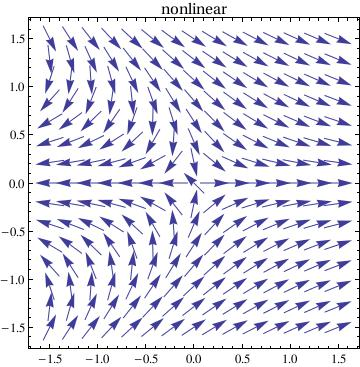Now we plot phase portrait for a linear system of ordinary differential equations A = {{1, 1}, {1, -1}}; ic = {{.5, .5}, {-.5, -.5}, {-.5, .5}, {.5, -.5}, {.1, 1.1}, {1.1, .1}, {-1.1, .1}, {0.1, -1.1}}; T = 10; sol = Table[NDSolve[{x'[t] == (A.{x[t], y[t]})[], y'[t] == (A.{x[t], y[t]})[], x == ic[[j, 1]], y == ic[[j, 2]]}, {x, y}, {t, 0, T}], {j, Length[ic]}]; solb = Table[ NDSolve[{x'[t] == (A.{x[t], y[t]})[], y'[t] == (A.{x[t], y[t]})[], x == ic[[j, 1]], y == ic[[j, 2]]}, {x, y}, {t, 0, -T}], {j, Length[ic]}]; p1 = Show[ ParametricPlot[ Table[{x[t], y[t]} /. sol[[j]], {j, Length[ic]}], {t, 0, T}, PlotRange -> {{-3, 3}, {-3, 3}}], ParametricPlot[ Table[{x[t], y[t]} /. solb[[j]], {j, Length[ic]}], {t, 0, -T}, PlotRange -> {{-3, 3}, {-3, 3}}], Table[Graphics[Arrow[{ic[[j]], ic[[j]] + .1 A.ic[[j]]}]], {j, Length[ic]}], PlotLabel -> Style[StandardForm["Phase Portrait"], FontSize -> 14], AxesLabel -> {Style[StandardForm["x"], FontSize -> 14], Style[StandardForm["y"], FontSize -> 14]}] Plot[{x[t] /. sol[], y[t] /. sol[]}, {t, 0, T}, PlotStyle -> {RGBColor[1, 0, 0], RGBColor[0, 1, 0]}, AxesOrigin -> {0, 0}, AxesLabel -> {"t", "x(t), y(t)"}, PlotLabel -> "Time courses", BaseStyle -> {FontFamily -> "Times", FontSize -> 14}]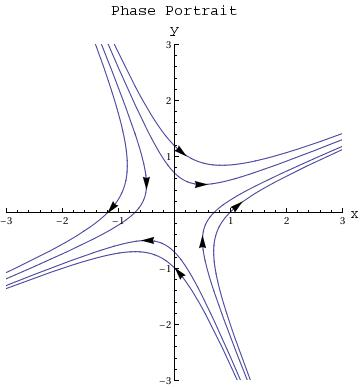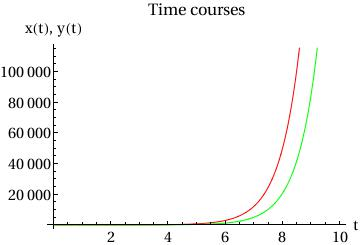Then we plot non-linear system along with the linear one (for comparison) ic = {{.5, .5}, {-.5, -.5}, {-.5, .5}, {.5, -.5}, {1, 1}, {1, -1}, {-1, 1}, {-1, -1}, {0, -.1}, {0, .1}, {-.2, -.2}, {-.2, .2}}; T = 5; sol = Table[NDSolve[{x'[t] == (A.{x[t], y[t]})[], y'[t] == (A.{x[t], y[t]})[], x == ic[[j, 1]], y == ic[[j, 2]]}, {x, y}, {t, 0, T}], {j, Length[ic]}]; solb = Table[ NDSolve[{x'[t] == (A.{x[t], y[t]})[], y'[t] == (A.{x[t], y[t]})[], x == ic[[j, 1]], y == ic[[j, 2]]}, {x, y}, {t, 0, -T}], {j, Length[ic]}]; solnonlin = Table[NDSolve[{x'[t] == f[x[t], y[t]][], y'[t] == f[x[t], y[t]][], x == ic[[j, 1]], y == ic[[j, 2]]}, {x, y}, {t, 0, T}], {j, Length[ic]}]; solnonlinb = Table[NDSolve[{x'[t] == f[x[t], y[t]][], y'[t] == f[x[t], y[t]][], x == ic[[j, 1]], y == ic[[j, 2]]}, {x, y}, {t, 0, -T}], {j, Length[ic]}]; xyr = 3; p1 = Show[ ParametricPlot[ Table[{x[t], y[t]} /. sol[[j]], {j, Length[ic]}], {t, 0, T}, PlotRange -> {{-xyr, xyr}, {-xyr, xyr}}], ParametricPlot[ Table[{x[t], y[t]} /. solb[[j]], {j, Length[ic]}], {t, 0, -T}, PlotRange -> {{-xyr, xyr}, {-xyr, xyr}}], Table[Graphics[{Arrowheads[.05], Arrow[{ic[[j]], ic[[j]] + .1 A.ic[[j]]}]}], {j, Length[ic]}], PlotLabel -> Style["Phase Portrait - Linearized", FontSize -> 20], AxesLabel -> {Style[StandardForm["x"], FontSize -> 14], Style[StandardForm["y"], FontSize -> 14]}]; p2 = Show[ ParametricPlot[ Table[{x[t], y[t]} /. solnonlin[[j]], {j, Length[ic]}], {t, 0, T}, PlotRange -> {{-xyr, xyr}, {-xyr, xyr}}], ParametricPlot[ Table[{x[t], y[t]} /. solnonlinb[[j]], {j, Length[ic]}], {t, 0, -T}, PlotRange -> {{-xyr, xyr}, {-xyr, xyr}}], Table[Graphics[{Arrowheads[.05], Arrow[{ic[[j]], ic[[j]] + .1 f[ic[[j, 1]], ic[[j, 2]]]}]}], {j, Length[ic]}], PlotLabel -> Style["Phase Portrait - Nonlinear", FontSize -> 20], AxesLabel -> {Style[StandardForm["x"], FontSize -> 14], Style[StandardForm["y"], FontSize -> 14]}]; p3 = GraphicsArray[{p2, p1}, ImageSize -> 600, Frame -> None]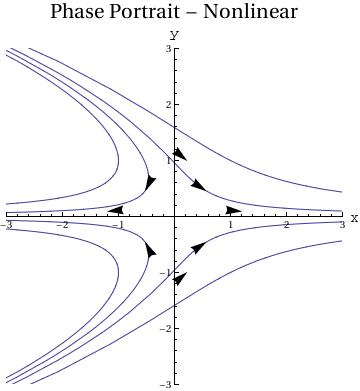f1 = 2*x - x*x - 3*x*y Out= 2 x - x^2 - 3 x y f2 = 3*y - 2*y*y - 4*x*y Out= 3 y - 2 y^2 - 4 x y s = Solve[{f1/x == 0, f2/y == 0}, {x, y}] Out= {{x -> 4/5, y -> 3/10}} df = {{D[f1, x], D[f1, y]}, {D[f2, x], D[f2, y]}} Out= {{2 - 2 x - 3 y, -3 x}, {-4 y, 3 - 4 x - 4 y}} A = df /. s Out= {{{-(1/2), -(3/2)}, {-2, -1}}} Eigenvalues[A] Out= {-(5/2), 1} ■ Linear Stability Analysis An equilibrium for the autonomous equation $$\dot{\bf x} = {\bf f}\left( {\bf x} \right) .$$ is the point x* where f(x*) = 0. This critical point x* is stable or unstable depending on the behavior of solutions of the vector differential equation $$\dot{\bf x} = {\bf f}\left( {\bf x} \right)$$ near the equilibrium. It is natural to assume that x(t) is near x* and as an approximation, we replace f(x) by its linearization with the Jacobian J evaluated at the critical point: \begin{equation} \label{EqLinear.9} \frac{{\text d}{\bf x}(t)}{{\text d}t} = {\bf J}\left( {\bf x}^{\ast} \right) \left( {\bf x} - {\bf x}^{\ast} \right) . \end{equation} The vector differential equation \eqref{EqLinear.9} simplifies the analysis of nonlinear system because it is a constant coefficient linear differential system with constant matrix J(x*). Upon introducing a new dependent variable, we reduce the problem to the case when the critical point is the origin: \begin{equation} \label{EqLinear.10} \frac{{\text d}{\bf y}(t)}{{\text d}t} = {\bf J}\left( {\bf x}^{\ast} \right) {\bf y} , \qquad {\bf J} = \texttt{D}{\bf f} = \left[ \partial f_i /\partial x_j \right] = \begin{bmatrix} \frac{\partial f_1}{\partial x_1} & \frac{\partial f_2}{\partial x_2} & \cdots & \frac{\partial f_2}{\partial x_n} \\ \frac{\partial f_2}{\partial x_1} & \frac{\partial f_2}{\partial x_2} & \cdots & \frac{\partial f_1}{\partial x_n} \\ \vdots & \vdots & \ddots & \vdots \\ \frac{\partial f_n}{\partial x_1} & \frac{\partial f_n}{\partial x_2} & \cdots & \frac{\partial f_n}{\partial x_n} \end{bmatrix} . \end{equation} The linearization theorem that shows equivalence of nonlinear system and its linearozed version is known as the Grobman--Hartman theorem. This theorem was first proved (Grobman, D. M. (1959). "О гомеоморфизме систем дифференциальных уравнений" [Homeomorphisms of systems of differential equations]. Doklady Akademii Nauk SSSR. 128: 880–881.) in 1959 by the Russian mathematician David Matveevich Grobman (born in 1922) from Moscow University, student of Nemytsckii. The next year, Philip Hartman (1915--2015) at John Hopkins University (USA) independently confirmed this result (Hartman, Philip (1960). "A lemma in the theory of structural stability of differential equations". Proceedings of the American Mathematical Society, 11, (4): 610–620. doi:10.2307/2034720). The theorem states that the behaviour of a dynamical system in a domain near a hyperbolic equilibrium point is qualitatively the same as the behaviour of its linearisation near this equilibrium point, where hyperbolicity means that no eigenvalue of the linearisation has real part equal to zero. In order to check this property, one may use the general Routh--Hurwitz condition: Consider the polynomial \[ \chi (\lambda ) = \lambda^n + a_{n-1} \lambda^{n-1} + \cdots + a_1 \lambda + a_0$
with real coefficients. With this polynomial, we associate the Hurwitz determinants:
$D_1 = a_{n-1} , \quad D_2 = \begin{vmatrix} a_{n-1} & 1 \\ a_{n-3} & a_{n-2} \end{vmatrix} , \quad D_3 = \begin{vmatrix} a_{n-1} & 1 & 0 \\ a_{n-3} & a_{n-2} & a_{n-1} \\ a_{n-5} & a_{n-4} & a_{n-3} \end{vmatrix} , \quad \ldots \quad D_n = \begin{vmatrix} a_{n-1} & 1 & 0 & \cdots & 0 \\ a_{n-3} & a_{n-2} & a_{n-1} & \cdots & 0 \\ \cdots & \cdots & \cdots & \cdots & \cdots \\ a_{n-5} & a_{n-4} & a_{n-3} & \cdots & a_0 \end{vmatrix} .$
Then a necessary and sufficient condition for all roots of the polynomial to lie in the left half complex plane Reλ < 0 is that Dk > 0 for all kn.
An equilibrium point is said to be hyperbolic if all the eigenvalues of its linearized system of equations has non-zero real part.
Theorem 2 (Lyapunov): 1. An equilibrium point in a nonlinear system is asymptotically Lyapunov stable if all the eigenvalues of the corresponding Jacobian have negative real parts.
2. An equilibrium point in a nonlinear system is Lyapunov unstable if there exists at least one eigenvalue of the corresponding Jacobian that has a positive real part.

Thus, Lyapunov’s theorems state that if the equilibrium is hyperbolic, then its linear approximations correctly predict the Lyapunov stability in the nonlinear system. (Note that in the second of Lyapunov’s theorems, it is not necessary for the equilibrium to be hyperbolic since the presence of an eigenvalue with positive real part implies instability even if it is accompanied by other eigenvalues with zero real part.)
Theorem 3: Consider a system
\begin{equation*} \dot{\bf x} = {\bf A}\, {\bf x}(t) + {\bf g}({\bf x}) , \qquad {\bf x}\in \mathbb{R}^n , \tag{2} \end{equation*}
where A is an n×n matrix and g is a continuous function having the property that for every γ>0 there exists δ(γ)>0 such that if $$\| {\bf x} \| < \delta (\gamma ) ,$$ then $$\| {\bf g}({\bf x}) \| \le \gamma \| {\bf x} \| .$$
Assume that all roots of the characteristic polynomial $$\chi (\lambda ) = \det \left( \lambda{\bf I} - {\bf A} \right)$$ satisfy indequalities ℜλk < 0,    k = 1, 2, … , n.
Then these exist δ0>0, β≥1 such that if $$\| {\bf x}_0 \| < \delta_0 ,$$ then it follows that
$\| {\bf x}(t; t_0 , {\bf x}_0 )\| \le \beta \,e^{-\alpha \left( t - t_0 \right) /2} \| {\bf x}_0 \| , \qquad t\ge t_0 .$
Here $${\bf x}(t; t_0 , {\bf x}_0 )$$ is the solution of Eq.(2) subject to the initial condition x(t0) = x0.

Proof: Let $${\bf x}(t; t_0 , {\bf x}_0 )$$ be a solution of Eq.(2). Then we have

$\frac{\text d}{{\text d}t}\,{\bf x}(t; t_0 , {\bf x}_0 ) = {\bf A}\,{\bf x}(t; t_0 , {\bf x}_0 ) + h(t), \qquad h(t) = {\bf g} \left( {\bf x}(t; t_0 , {\bf x}_0 ) \right) .$
By using the variation of parameters formula, we get
${\bf x}(t; t_0 , {\bf x}_0 ) = e^{{\bf A} \left( t - t_0 \right)} {\bf x}_0 + \int_{t_0}^t e^{{\bf A} \left( t - s \right)} h(s) \,{\text d}s ,$
that is,
${\bf x}(t; t_0 , {\bf x}_0 ) = e^{{\bf A} \left( t - t_0 \right)} {\bf x}_0 + \int_{t_0}^t e^{{\bf A} \left( t - s \right)} {\bf g} \left( {\bf x}(s; t_0 , {\bf x}_0 ) \right) {\text d}s .$
The assumption concerning the roots of the characteristic polynomial implies that there exists β≥1 such that $$\displaystyle \left\| e^{{\bf A} \left( t - s \right)} \right\| \le \beta \, e^{-\alpha \left( t-s \right)}$$ for all ts. From this inequality, we deduce
$\| {\bf x}(t; t_0 , {\bf x}_0 ) \| \le \beta\, e^{-\alpha \left( t - t_0 \right)}\| {\bf x}_0 \| + \int_{t_0}^t \beta \,e^{-\alpha \left( t - s \right)} \| {\bf g} \left( {\bf x}(s; t_0 , {\bf x}_0 ) \right) \| \,{\text d}s .$
We take γ = α/(2β), δ0 = δ(γ)/(2β), and assume that $$\| {\bf x}_0 \| < \delta_0 .$$ Then there exists a maximal interval [t0, t0 + T) such that $$\| {\bf x}(t; t_0 , {\bf x}_0 ) \| < \delta (\gamma )$$ for all t ∈ [t0, t0 + T), For such t, we have $$\| {\bf g} \left( {\bf x}(s; t_0 , {\bf x}_0 ) \right) \| \le \gamma\,\| {\bf x}(s; t_0 , {\bf x}_0 ) \|, \quad t_0 \le s \le t .$$ Hence
$\| {\bf x}(t; t_0 , {\bf x}_0 ) \| \le \beta\, e^{-\alpha \left( t - t_0 \right)}\| {\bf x}_0 \| + \beta\gamma \int_{t_0}^t e^{-\alpha \left( t - s \right)} \| \left( {\bf x}(s; t_0 , {\bf x}_0 ) \| {\text d}s$
or
$e^{\alpha t} \| {\bf x}(t; t_0 , {\bf x}_0 ) \| \le \beta\, e^{\alpha t_0} \| {\bf x}_0 \| + \beta\gamma \int_{t_0}^t e^{\alpha s} \| {\bf x}(s; t_0 , {\bf x}_0 ) \| {\text d}s$
$e^{\alpha t} \| {\bf x}(t; t_0 , {\bf x}_0 ) \| \le \beta\, e^{\alpha t_0} \| {\bf x}_0 \| e^{\beta\gamma \left( t - t_0 \right)} ,$
that is,
$\| {\bf x}(t; t_0 , {\bf x}_0 ) \| \le \beta\, e^{- \left( \alpha - \beta\gamma \right) \left( t - t_0 \right)} \| {\bf x}_0 \| < \beta \,e^{-\alpha \left( t - t_0 \right) /2} \| {\bf x}_0 \|$
because 2βγ < α.

The inequality above holds for t0t < t0 + T for finite T. Then we would have
﹡ ⁎ ✱ ✲ ✳ ✺ ✻ ✼ ✽ ❋

Example 3: Consider the nonlinear system
$\frac{{\text d}x}{{\text d}t} = - x - \frac{y}{\ln \sqrt{x^2 + y^2}} , \qquad \frac{{\text d}y}{{\text d}t} = - y + \frac{x}{\ln \sqrt{x^2 + y^2}}$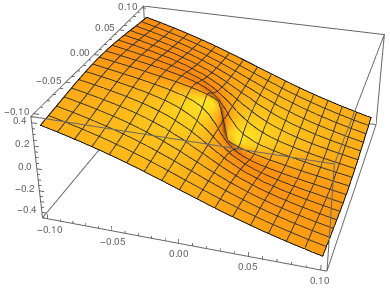The given nonlinear system of differential equations reseambles an almost linear system because the nonlinear functions in the right-hand side tends to zero faster than ∥x∥. However, the functions $g_1(x,y) = - \frac{y}{\ln \sqrt{x^2 + y^2}} , \qquad g_2 (x,y) = \frac{x}{\ln \sqrt{x^2 + y^2}}$ are not holomorphic at the origin. So they cannot be used in Grobman--Hartman theorem. The 3D plot of $$g_1 (x,y) /\sqrt{x^2 + y^2}$$ shows that the ratio tends to zero near the origin. f[x_, y_] = x/Sqrt[x^2 + y^2]/Log[Sqrt[x^2 + y^2]]; Plot3D[f[x, y], {x, -0.1, 0.1}, {y, -0.1, 0.1}, ClippingStyle -> Opacity[0.6]] Plot of the nonlinear function devided by ∥x∥. Mathematica code

The polar equations corresponding to the given nonlinear system are
$\frac{{\text d}r}{{\text d}t} = -r, \qquad \frac{{\text d}\theta}{{\text d}t} = \frac{1}{\ln r}$
Its solution is
$r = \rho (t) = c\,e^{-t} , \qquad \theta = \omega (t) = - \ln \left( t- \ln c \right) + k$
for some positive constant c and k = ω(t0) + ln(t0 - lnc). We consclude that
$\omega (t) \to -\infty \qquad\mbpx{as} \quad t \to +\infty .$
Therefore, the origin is a spiral point for the given nonlinear system although the origin is a proper node to the linear system
$\frac{{\text d}x}{{\text d}t} = -x, \qquad \frac{{\text d}y}{{\text d}t} = -y .$
The following direction fields show that the linear approximation does not resemble the nonlinear case.
treamPlot[{-x - y/Log[Sqrt[x^2 + y^2]], -y + x/Log[Sqrt[x^2 + y^2]]}, {x, -1, 1}, {y, -1, 1}, PlotTheme -> "Scientific", StreamStyle -> Blue, PerformanceGoal -> "Quality", StreamPoints -> 25]
StreamPlot[{-x, -y}, {x, -2, 2}, {y, -2, 2}, PerformanceGoal -> "Quality", PlotTheme -> "Web"]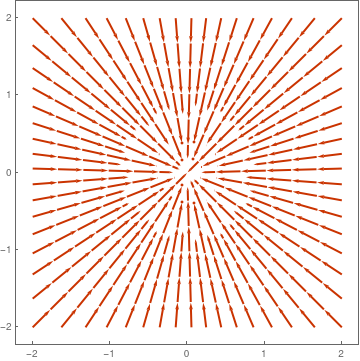Phase portrait for the nonlinear system. Phase portrate for linear part.

■
Example 4: Consider the nonlinear system
$\frac{{\text d}x}{{\text d}t} = - x +y + \frac{x}{\ln \sqrt{x^2 + y^2}} , \qquad \frac{{\text d}y}{{\text d}t} = -x- y + \frac{y}{\ln \sqrt{x^2 + y^2}}$
The polar equations corresponding to the given nonlinear system are
$r^{-1}\frac{{\text d}r}{{\text d}t} = -1 + \frac{1}{\ln r}, \qquad \frac{{\text d}\theta}{{\text d}t} = \frac{1}{\ln r}$
Its solution r = ρ(t) is defined implicitly
$\rho (t)\,e^t = \frac{\rho_0}{\ln \rho (t) -1} ,$
where ρ0 is a constant.

Therefore,

$\rho (t)\, e^t \to 0 \qquad \mbox{as} \quad t\to \infty .$
and the origin is not a proper spiral for the nonlinear system of differential equations, although (0,0) is a proper spiral for its linear approximation
$\begin{cases} \dot{x} = -x+y \\ \dot{y} = -x-y . \end{cases}$
So the center is the critical point for the linear system, but nonlinear one has the piral.

The following direction fields show that the linear approximation does not match the nonlinear case.
StreamPlot[{-x + y + x/Log[Sqrt[x^2 + y^2]], -x - y + y/Log[Sqrt[x^2 + y^2]]}, {x, -1, 1}, {y, -1, 1}, PlotTheme -> "Scientific", StreamStyle -> Blue, PerformanceGoal -> "Quality", StreamPoints -> 30]
StreamPlot[{-x + y, -x - y}, {x, -2, 2}, {y, -2, 2}, PerformanceGoal -> "Quality", PlotTheme -> "Web"]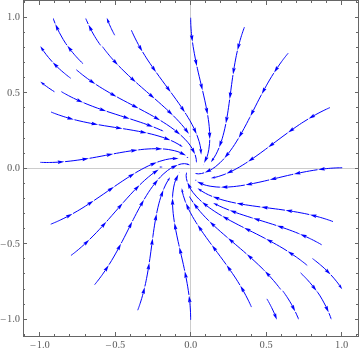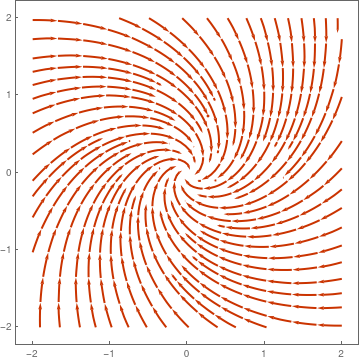Phase portrait for the nonlinear system. Phase portrate for linear part.

■

1. Mandelzweig, F. and Tabakin, F., Quasilinearization approach to nonlinear problems in physics with application to nonlinear ODEs, Comput. Phys.Commun. 141(2001)268.
2. Ramos, J.L., Linearization method in classical and quantum mechanics , Comput. Phys.Commun. 153(2003)199.